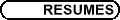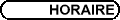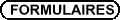SS10 - Analyse stochastique / SS10 - Stochastic Analysis
Org: M. Barlow (UBC) et/and D. Bakry (Toulouse)

MICHEL BENAIM, Neuchatel

NINA GANTERT, Inst. Math. Stochastik, Universitaet Karlsruhe
Deviations of random walk in random scenery

Let (Zn)n Î N0 be a d-dimensional random walk in random scenery, i.e., Zn = åk=0n-1 Y(Sk) with (Sk)k Î N0 a random walk in Zd and ( Y(z) )z Î Zd an i.i.d. scenery, independent of the walk. The walker's steps have mean zero and finite variance.

We identify the speed and the rate of the logarithmic decay of P([ 1/(n)] Zn > bn) for various choices of sequences (bn)n in [1,¥). Depending on (bn)n and the upper tails of the scenery, we identify different regimes for the speed of decay and different variational formulas for the rate functions. In contrast to recent work by A. Asselah and F. Castell, we consider sceneries unbounded to infinity. It turns out that there are interesting connections to large deviation properties of self-intersections of the walk, which have been studied recently by X. Chen.

RICHARD KENYON, CNRS / Paris Sud

VLADA LIMIC, University of British Columbia
Attracting edge in some strongly reinforced walks

Reinforcement is observed frequently in nature and society, where beneficial interactions tend to be repeated. Edge reinforced random walker on a graph remembers the number of times each edge was traversed in the past, and decides to make the next random step with probabilities favouring places visited before. Using martingale techniques and comparison with the generalized Urn scheme, it is shown in  that the edge reinforced random walker on a graph of bounded degree, with the reinforcement weight function W(k) = kr, r > 1, traverses a random attracting edge at all large times, with probability 1. A remarkably short argument of Sellke  shows that attracting edge exists if and only if

 å k 1 W(k) < ¥, \leqno(1)
whenever the underlying graph has no odd cycle. The conjecture that condition (1) implies existence of attracting edge when the underlying graph is a triangle is still open.

Progress has been made recently  towards better understanding of attracting edge property for convex and increasing weights W with property (1).

References


V. Limic, Attracting edge property for a class of reinforced random walks. Ann. Probab. 31(2003), 1615-1654.


T. Sellke, Reinforced random walks on the d-dimensional integer lattice. Preprint, 1994.


V. Limic and P. Tarrès, in progress.

PIERRE MATHIEU, CMI Université de Provence
Centered Markov chains

A reversible measure turns a Markov operator into a symmetric operator and spectral theory can then be used to study the transition kernel. We introduce the more general notion of `centered measure' and prove an upper estimate on the decay of the transition probabilities of Carne-Varopoulos type. The connection with the rate of escape in the case of random walks on discrete groups will be discussed.

PIERRE TARRES, Université Paul Sabatier, Toulouse, and Université de Neuchâtel
Self-Interacting Random Walks

A self-interacting random walk is a random process evolving in an environment depending on its past behavior.

The notion of Edge-Reinforced Random Walk (ERRW) was introduced in 1986 by Coppersmith and Diaconis  on a discrete graph, with the probability of a move along an edge being proportional to the number of visits to this edge. In the same spirit, Pemantle introduced in 1988  the Vertex-Reinforced Random Walk (VRRW), the probability of a move to an adjacent vertex being then proportional to the number of visits to this vertex (and not to the edge leading to the vertex). The Self-Interacting Diffusion (SID) is a continuous counterpart to these notions.

Although introduced by similar definitions, these processes show some significantly different behaviors, leading in their understanding to various methods. While the study of ERRW essentially requires some probabilistic tools, corresponding to some local properties, the comprehension of VRRW and SID needs a joint understanding of on one hand a dynamical system governing the general evolution, and on the other hand some probabilistic phenomena, acting as a perturbation, and sometimes changing the nature of this dynamical system.

The purpose of our talk is to present our recent results on the subject , , , .

References


M. Benaïm and P. Tarrès, Dynamics of vertex-reinforced random walks. In progress, 2004.


D. Coppersmith and P. Diaconis, Random walks with reinforcement. Unpublished manuscript, 1986.


V. Limic and P. Tarrès. In progress, 2004.


T. Mountford and P. Tarrès, An asymptotic result for brownian polymers. Preprint, 2004.


R. Pemantle, Random processes with reinforcement. MIT Doctoral Dissertation, 1988.


P. Tarrès, VRRW on Z eventually gets stuck on five points. Annals of Probability, 2004, to appear.